Latest Questions UpdateCategory: MathematicsNewton Raphson Method with example, Engineering Mathematics
Using the method of Newton Raphson, find the real roots of the equation x3 – 3x – 5 = 0 correct to four places of decimals. This question comes from Numerical Analysis, Engineering Book.
To find the roots of the equation x3 – 3x – 5 up to 5 decimal places using the Newton Raphson Method. Follow the steps to solve the questions.

Given equation f(x) = x3 – 3x – 5

Differentiate with respect to x, we get f ‘(x) = 3x2 – 3

Now, first find the range, where the real roots lie in i.e. f(2) = –3 and f(3) = 13

Since f(2) is a negative value and f(3) is a positive value. Therefore, our one real root of the equation lies between x = 2 and 3.

Now, by Newton-Raphson formula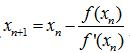Now, substitute the value of f(x) and f'(x) in this formula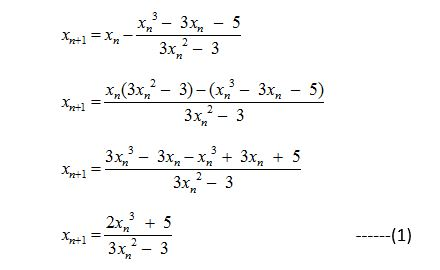Choose the initial approximate value of the root x0 = 2 and putting n = 0, we have the first approximate value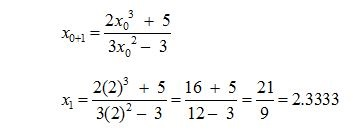Now, for the second approximation, replacing n = 1 in equation (1) we get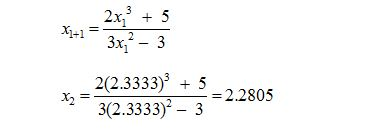Now, for the third approximation, replacing n = 2 in equation (1) we get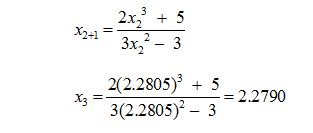Now, for the Fourth approximation, replacing n = 3 in equation (1) we get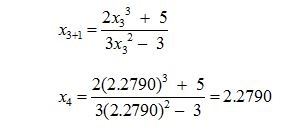Here x3 and x4 obtained the same value; therefore one root of the given equation = x3 – 3x – 5 using the Newton Raphson Method is 2.2790.

Read More Articles – See tips by experts

1. How to get Maximum Marks in Examination Preparation Strategy by Dr. Mukesh Shrimali
2. Physics & Maths tutor helps to improve your child’s knowledge
3. 5 Important tips to Personal Development apply in your daily life
4. Reasons to attempt ONLINE MOCK TEST SERIES for Bank PO Exams